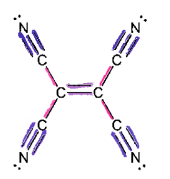# Problem: The total number of sigma and pi bonds in the following molecule are:  Sigma bonds:______ Pi bonds: ________

🤓 Based on our data, we think this question is relevant for Professor Sanders' class at QC CUNY .

###### Problem Details

The total number of sigma and pi bonds in the following molecule are:

Sigma bonds:______
Pi bonds: ________What scientific concept do you need to know in order to solve this problem?

Our tutors have indicated that to solve this problem you will need to apply the Orbital Overlap concept. If you need more Orbital Overlap practice, you can also practice Orbital Overlap practice problems.

What is the difficulty of this problem?

Our tutors rated the difficulty ofThe total number of sigma and pi bonds in the following mole...as medium difficulty.

How long does this problem take to solve?

Our expert Chemistry tutor, Sabrina took 2 minutes and 13 seconds to solve this problem. You can follow their steps in the video explanation above.

What professor is this problem relevant for?

Based on our data, we think this problem is relevant for Professor Sanders' class at QC CUNY .